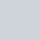# C++ Program to Find Out Sum of Digits

Last updated on:

In this tutorial, we will learn about the sum of digits in C++ i.e. how to write a program find out the sum of digits of any number using C++.

## Quick Info:💡

↪ A program to compute the sum of digits in an integer.

↪ For example, 345=3+4+5=12

↪ Steps to find out the sum of digits:

• Get any number by the user
• Get the remainder of the number
• Find the sum of a remainder
• Divide the number by 10
• Repeat above steps till number becomes less than 0

## Find Out Sum of Digits Using While loop

For finding the sum of digits, we will try to execute the above steps with the help of C++ programming language.

Here’s a direct and simple method to find out sum of digits.

Simply, the user will be asked to enter an integer then, the number is computed according to code provided and then the result is displayed to a user.

### Example:

``````#include<iostream.h>
#include<conio.h>
void main()
{
int num, sum=0, rem;
cout<<"Enter any number:=";
cin>>num;
while(num>0)
{
rem=num%10;
sum=sum+rem;
num=num/10;
}
cout<<"Sum of digits:="<<sum;
getch();
}``````
``````//Output:
Enter any number:=3456
Sum of digits:=18``````

### Working:

Flow of a program:

Above figure is the working explanation of the program.

And, you can also try this program(i.e. sum of digits) using the function.

Some other programs that can help you to write a code using the function: Palindrome, Reverse or Armstrong.

Well, I hope it really helped you to know C++ better.

Attention reader⚠ Don’t stop learning now.

Just stay with us to get more such exciting codes to explore more in C++.

1.